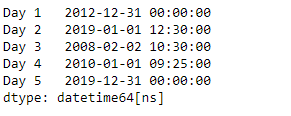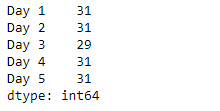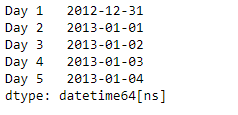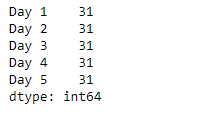# Python | Pandas Series.dt.days_in_month

`Series.dt` can be used to access the values of the series as datetimelike and return several properties. Pandas` Series.dt.days_in_month` attribute return the number of days in the month for the given series object.

Syntax: Series.dt.days_in_month

Parameter : None

Returns : numpy array

Example #1: Use `Series.dt.days_in_month` attribute to find the number of days in the month of the given date in series object.

 `# importing pandas as pd ` `import` `pandas as pd ` ` `  `# Creating the Series ` `sr ``=` `pd.Series([``'2012-12-31'``, ``'2019-1-1 12:30'``, ``'2008-02-2 10:30'``, ` `               ``'2010-1-1 09:25'``, ``'2019-12-31 00:00'``]) ` ` `  `# Creating the index ` `idx ``=` `[``'Day 1'``, ``'Day 2'``, ``'Day 3'``, ``'Day 4'``, ``'Day 5'``] ` ` `  `# set the index ` `sr.index ``=` `idx ` ` `  `# Convert the underlying data to datetime  ` `sr ``=` `pd.to_datetime(sr) ` ` `  `# Print the series ` `print``(sr) `

Output :Now we will use `Series.dt.days_in_month` attribute to find the number of days in the month for the given date.

 `# find the number of  ` `# days in the month ` `result ``=` `sr.dt.days_in_month ` ` `  `# print the result ` `print``(result) `

Output :As we can see in the output, the `Series.dt.days_in_month` attribute has successfully accessed and returned the number of days in the month for the given date.

Example #2 : Use `Series.dt.days_in_month` attribute to find the number of days in the month of the given date in series object.

 `# importing pandas as pd ` `import` `pandas as pd ` ` `  `# Creating the Series ` `sr ``=` `pd.Series(pd.date_range(``'2012-12-31 00:00'``, periods ``=` `5``, freq ``=` `'D'``)) ` ` `  `# Creating the index ` `idx ``=` `[``'Day 1'``, ``'Day 2'``, ``'Day 3'``, ``'Day 4'``, ``'Day 5'``] ` ` `  `# set the index ` `sr.index ``=` `idx ` ` `  `# Print the series ` `print``(sr) `

Output :Now we will use `Series.dt.days_in_month` attribute to find the number of days in the month for the given date.

 `# find the number of  ` `# days in the month ` `result ``=` `sr.dt.days_in_month ` ` `  `# print the result ` `print``(result) `

Output :As we can see in the output, the `Series.dt.days_in_month` attribute has successfully accessed and returned the number of days in the month for the given date.

My Personal Notes arrow_drop_upCheck out this Author's contributed articles.

If you like GeeksforGeeks and would like to contribute, you can also write an article using contribute.geeksforgeeks.org or mail your article to contribute@geeksforgeeks.org. See your article appearing on the GeeksforGeeks main page and help other Geeks.

Please Improve this article if you find anything incorrect by clicking on the "Improve Article" button below.

Article Tags :

Be the First to upvote.

Please write to us at contribute@geeksforgeeks.org to report any issue with the above content.